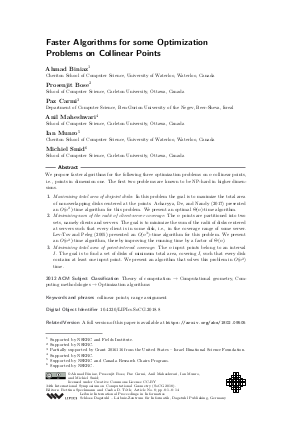Document# Faster Algorithms for some Optimization Problems on Collinear Points

### Authors Ahmad Biniaz, Prosenjit Bose, Paz Carmi, Anil Maheshwari, Ian Munro, Michiel Smid## File

LIPIcs.SoCG.2018.8.pdf
• Filesize: 0.78 MB
• 14 pages

## Cite As

Ahmad Biniaz, Prosenjit Bose, Paz Carmi, Anil Maheshwari, Ian Munro, and Michiel Smid. Faster Algorithms for some Optimization Problems on Collinear Points. In 34th International Symposium on Computational Geometry (SoCG 2018). Leibniz International Proceedings in Informatics (LIPIcs), Volume 99, pp. 8:1-8:14, Schloss Dagstuhl - Leibniz-Zentrum für Informatik (2018)
https://doi.org/10.4230/LIPIcs.SoCG.2018.8

## Abstract

We propose faster algorithms for the following three optimization problems on n collinear points, i.e., points in dimension one. The first two problems are known to be NP-hard in higher dimensions. 1) Maximizing total area of disjoint disks: In this problem the goal is to maximize the total area of nonoverlapping disks centered at the points. Acharyya, De, and Nandy (2017) presented an O(n^2)-time algorithm for this problem. We present an optimal Theta(n)-time algorithm. 2) Minimizing sum of the radii of client-server coverage: The n points are partitioned into two sets, namely clients and servers. The goal is to minimize the sum of the radii of disks centered at servers such that every client is in some disk, i.e., in the coverage range of some server. Lev-Tov and Peleg (2005) presented an O(n^3)-time algorithm for this problem. We present an O(n^2)-time algorithm, thereby improving the running time by a factor of Theta(n). 3) Minimizing total area of point-interval coverage: The n input points belong to an interval I. The goal is to find a set of disks of minimum total area, covering I, such that every disk contains at least one input point. We present an algorithm that solves this problem in O(n^2) time.
##### Keywords
• collinear points
• range assignment

## Metrics

• Access Statistics
• Total Accesses (updated on a weekly basis)
0

## References

1. Ankush Acharyya, Minati De, and Subhas C. Nandy. Range assignment of base-stations maximizing coverage area without interference. In Proceedings of the 29th Canadian Conference on Computational Geometry (CCCG), pages 126-131, 2017.2. Ankush Acharyya, Minati De, Subhas C. Nandy, and Bodhayan Roy. Range assignment of base-stations maximizing coverage area without interference. CoRR, abs/1705.09346, 2017.3. Helmut Alt, Esther M. Arkin, Hervé Brönnimann, Jeff Erickson, Sándor P. Fekete, Christian Knauer, Jonathan Lenchner, Joseph S. B. Mitchell, and Kim Whittlesey. Minimum-cost coverage of point sets by disks. In Proceedings of the 22nd ACM Symposium on Computational Geometry, (SoCG), pages 449-458, 2006.4. Vittorio Bilò, Ioannis Caragiannis, Christos Kaklamanis, and Panagiotis Kanellopoulos. Geometric clustering to minimize the sum of cluster sizes. In Proceedings of the 13th European Symposium on Algorithms, (ESA), pages 460-471, 2005.5. Ahmad Biniaz, Prosenjit Bose, Paz Carmi, Anil Maheshwari, Ian Munro, and Michiel Smid. Faster algorithms for some optimization problems on collinear points. CoRR, abs/1802.09505, 2018.6. Paz Carmi, Matthew J. Katz, and Joseph S. B. Mitchell. The minimum-area spanning tree problem. Computational Geometry: Theory and Applications, 35(3):218-225, 2006.7. Erin W. Chambers, Sándor P. Fekete, Hella-Franziska Hoffmann, Dimitri Marinakis, Joseph S. B. Mitchell, Srinivasan Venkatesh, Ulrike Stege, and Sue Whitesides. Connecting a set of circles with minimum sum of radii. Computational Geometry: Theory and Applications, 68:62-76, 2018.8. David Eppstein. Maximizing the sum of radii of disjoint balls or disks. In Proceedings of the 28th Canadian Conference on Computational Geometry (CCCG), pages 260-265, 2016.9. Ju Yuan Hsiao, Chuan Yi Tang, and Ruay Shiung Chang. An efficient algorithm for finding a maximum weight 2-independent set on interval graphs. Information Processing Letters, 43(5):229-235, 1992.10. Nissan Lev-Tov and David Peleg. Polynomial time approximation schemes for base station coverage with minimum total radii. Computer Networks, 47(4):489-501, 2005.X

Feedback for Dagstuhl Publishing